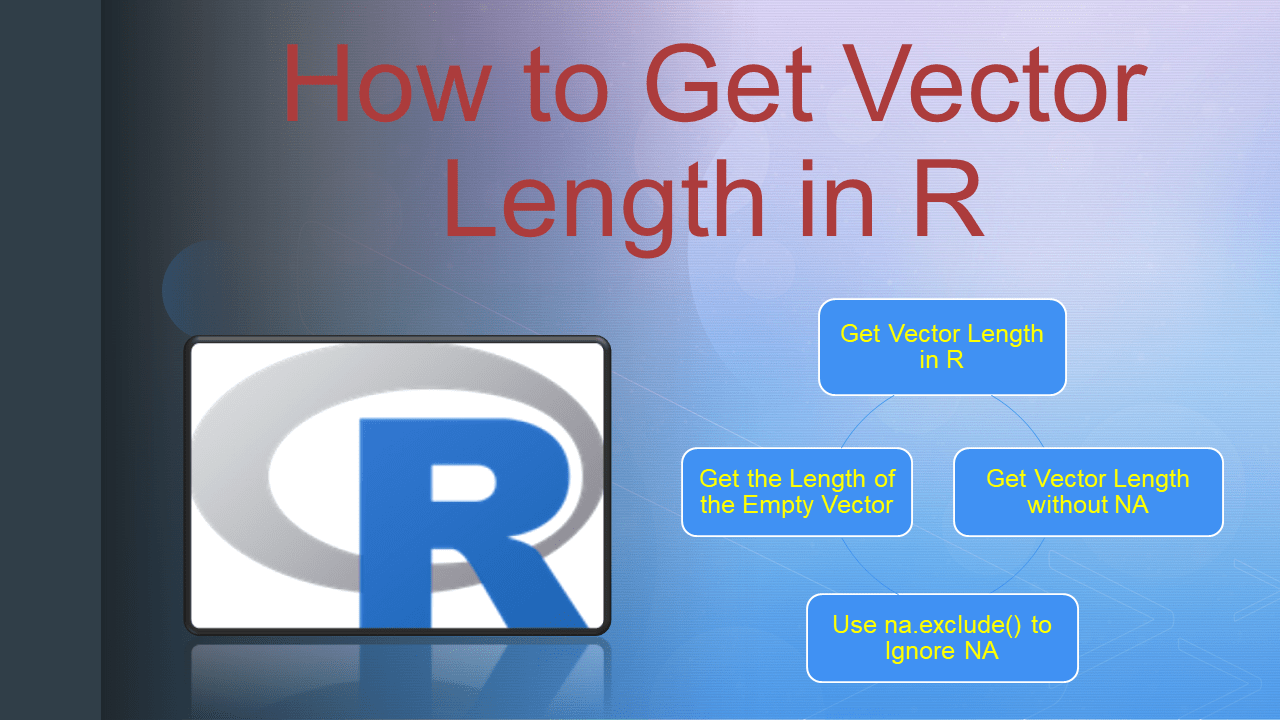# How to Get Vector Length in R?

To get the size (number of elements) of a vector in R use the `length()` function. This `length()` function returns the number of elements in a vector without ignoring NA values. To get the size without NA values use `na.omit()` and `na.exclude()` functions.

NA in R is considered a missing value.

## 1. Quick Examples of Vector Length

Following are quick examples of how to get the number of elements in R Vector.

``````
# Quick Examples

# Get Vector length
vec <- c('java','r',NA,'python')
length(vec)

# Get Vector Length without NA
vec <- c('java','r',NA,'python')
length(na.omit(vec))

# Get Vector Length without NA
vec <- c('java','r',NA,'python')
length(na.exclude(vec))

# Get length of empty vector
vec <- character()
length(vec)
``````

## 2. Get Vector Length in R

The `length()` is an R base function that is used to get the size or number of elements in a vector object. This function takes the vector as an argument for which you wanted to calculate the size and returns the length. The default method for `length()` returns a non-negative `integer` value.

Here, `c()` is used to create a vector in R.

``````
# Get lenght of vector
vec <- c('java','r',NA,'python')
length(vec)

# Output
4
``````

## 3. Get Vector Length without NA

If you have NA values in the R vector, the `length()` function considers the NA into the count. However, if you wanted to remove NA from vector before length() use `na.omit()`.

`na.omit()` function takes the vector as an argument and returns a vector by removing NA values, use this result on length() function to get the vector length without NA values.

``````
# Get Vector Length without NA
vec <- c('java','r',NA,'python')
length(na.omit(vec))

# Output
# 3
``````

## 4. Use na.exclude() to Ignore NA

Similarly, you can also use `na.exclude()` function to ignore NA values from the vector length.

``````
# Get Vector Length without NA
vec <- c('java','r',NA,'python')
length(na.exclude(vec))

# Output
# 3
``````

## 5. Get the Length of the Empty Vector

If you have an empty vector, the `length()` function returns 0 as the number of elements in a vector.

``````
# Get length of empty vector
vec <- character()
length(vec)

# Output
# 0
``````

## 6. Conclusion

In this article, you have learned how to get the size of the vector in R by using the length() function and also learned how to get length without NA by using na.omit() and na.exclude() functions. These na.omit() and na.exclude() functions take a vector as input and return by removing all NA values and use the result to length() function to get length without NA. You can find the complete example from this article at Github R Programming Examples Project.

## References

### Naveen (NNK)

Naveen (NNK) is a Data Engineer with 20+ years of experience in transforming data into actionable insights. Over the years, He has honed his expertise in designing, implementing, and maintaining data pipelines with frameworks like Apache Spark, PySpark, Pandas, R, Hive and Machine Learning. Naveen journey in the field of data engineering has been a continuous learning, innovation, and a strong commitment to data integrity. In this blog, he shares his experiences with the data as he come across. Follow Naveen @ @ LinkedIn